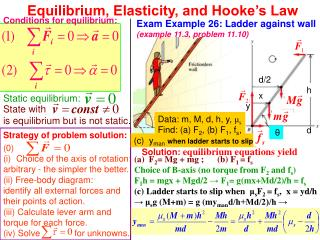Download PresentationEquilibrium, Elasticity, and Hooke’s Law

# Equilibrium, Elasticity, and Hooke’s Law

Download Presentation## Equilibrium, Elasticity, and Hooke’s Law

- - - - - - - - - - - - - - - - - - - - - - - - - - - E N D - - - - - - - - - - - - - - - - - - - - - - - - - - -
##### Presentation Transcript

1. Equilibrium, Elasticity, and Hooke’s Law Conditions for equilibrium: Exam Example 26: Ladder against wall(example 11.3, problem 11.10) d/2 h x Static equilibrium: y State with is equilibrium but is not static. Data: m, M, d, h, y, μs Find: (a) F2, (b) F1, fs, d θ Strategy of problem solution: (c) ymanwhen ladder starts to slip (0) • Choice of the axis of rotation: arbitrary - the simpler the better. (ii) Free-body diagram: identify all external forces and their points of action. (iii) Calculate lever arm and torque for each force. (iv) Solve for unknowns. Solution: equilibrium equations yield (a) F2= Mg + mg ; (b) F1 = fs Choice of B-axis (no torque from F2 and fs) F1h = mgx + Mgd/2 → F1= g(mx+Md/2)/h = fs (c) Ladder starts to slip when μsF2 = fs, x = yd/h → μsg (M+m) = g (mymand/h+Md/2)/h →

2. Center of Gravity Definition.The center of gravity of a rigid body is the point at which its weight can be considered to act when calculating the torque due to gravity: Derivation: (if has the same value at all points on a body) Center of gravity can be located by suspending object from different points. Note: Center of gravity may lie “outside” the object.

3. The larger the area of support and the lower the center of gravity, the more difficult it is to overturn a body.

4. Stress, Strain, and Elastic Moduli Stress = (Elastic modulus) · Strain Units: [stress F/A]=[pressure p] = 1 N/m2 = 1 pascal = 1 Pa = 1.45·10-4 psi (lb/in2) Young’s modulus Y~1011 N/m2 Shear modulus S~Y/3 Bulk modulus B~Y (only for solids, S=0 for fluids)

5. Stress versus Strain and Hooke’s Law Stress = (Elastic modulus) · Strain F = - kx Robert Hooke (1635-1703) (a contemporary of Newton) Elastic hysteresis

6. Interesting property: shorter springs are stiffer springs Proof: Force F is constant along the spring and x = x/2 + x/2 → F = - kx = - 2k (x/2) L+x L/2+x/2 L/2+x/2